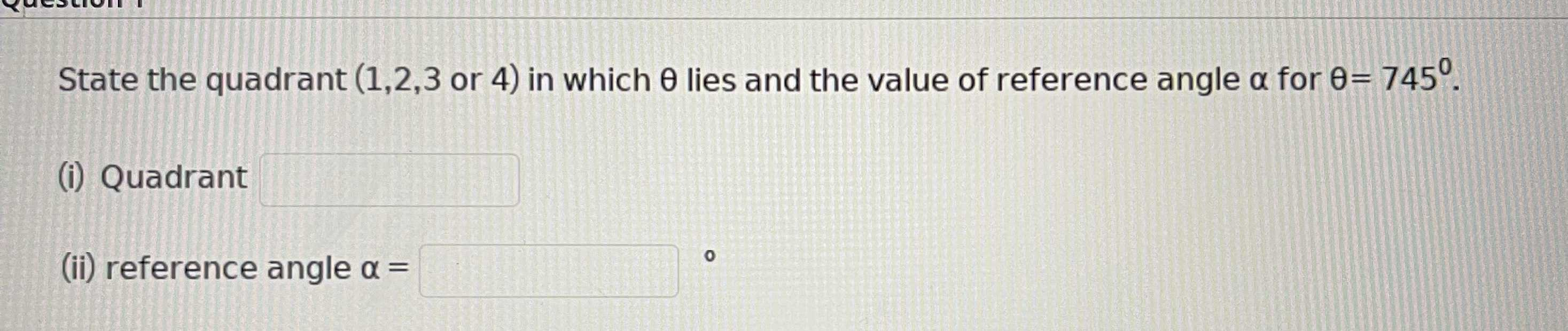### Still have math questions?

Trigonometry
QuestionState the quadrant $$( 1,2,3$$ or $$4 )$$ in which $$\theta$$ lies and the value of reference angle $$\alpha$$ for$$\theta = 745 ^ { \circ } .$$

(ii) reference angle $$\alpha =$$ _____
$$1\\ 25\degree$$